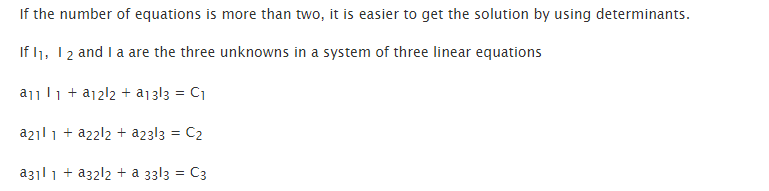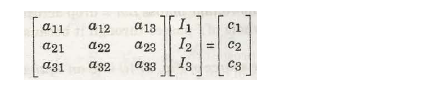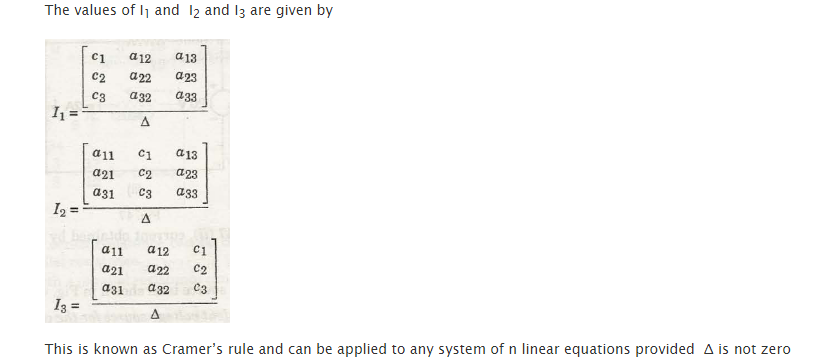Method of Determinants is considered as an easier solution when you have more than two equations to solve:

Let’s consider mentioned three equations:Where I1, I2 and I3 are the unknown, we need to solve the equation for.

Let’s write the same in the matrix form:We can calculate the value of the unknowns, I1, I2 and I3 by the following matrix calculation:Considering the value of △ as a non-zero figure, this rule can be applied to any system that has n number of linear equations. This rule is known as Cramer’s Rule.

Links of Next Electrical Engineering Topics:-### Customer Reviews

My Homework Help
Rated 5.0 out of 5 based on 510 customer reviews at